### SATELLITE BREAKUP ANALYSIS

#### HYPERVELOCITY COLLISIONS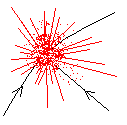Collisions between objects in space are invariably hypervelocity collisions. Such a collision is defined as one where the velocity (v) of the impactor (relative to the target) is so great that its kinetic energy ( K = ½ m v2) is greater than the energy released in the detonation of the same mass (m) of high explosive.

Given that the energy density of a typical high explosive (TNT) is 4.2 x 106 Joule/kg, we can compute the transition to hypervelocity from:

½ m v2 = 4.2 x 106 m
which gives us v ~ 3 km/sec. It is thus usual to regard a hypervelocity collision as one in which the relative velocity between target and impactor is 4 km/sec or greater.

In low Earth orbit, the velocity of a satellite is between 7 and 8 km/sec, and the typical collision velocity between two space objects in LEO is around 10 km/sec. Thus most accidental LEO collisions are hypervelocity collisions. The deliberate destruction of a satellite with a terrestrially launched missile will also be a hypervelocity collision. The missile does not need to carry an explosive warhead, as the destruction from the kinetic impact is greater than what could be achieved by high explosive.

A hypervelocity collision may be categorised as catastrophic or non-catastrophic. A catastrophic impact is one in which both the target and the impactor are totally destroyed, producing a range of fragments. In a non-catastrophic impact the impactor is detroyed and the target is damaged (or cratered), but not totally destroyed. Hypervelocity experiments have shown that target destruction usually results when the impactor kinetic energy is greater than 40,000 Mt Joule, where Mt is the mass of the target. If we designate the mass and relative velocity of the impactor as Mi and v respectively, we can calculate the ratio of target to impactor mass (the factor Γ = Mt / Mi) which divides the two classes of collision behavior:

Γ = v2 / 80000
For a relative collision velocity of 10 km/sec, Γ ~ 1250. Other data indicate that Γ may be as high as 2500 for a catastrophic collision.

Non-Catastrophic Collision

For this case:

Mi < Mt / Γ
In this case the impactor is totally destroyed and the ejected mass Me is given by:
Me = γ Mi
where experiment has shown that γ ~ 120 for collision velocities around 10 km/sec.

Catastrophic Collision

For a catastrophic collision:

Mi ≥ Mt / Γ
Both the impactor and the target are totally destroyed and the ejected mass is the sum of the two masses:
Me = Mi + Mt
In many cases the impactor mass is such a small fraction of the target mass that the ejected mass is essentially the target mass.

Fragment Mass Distribution

A simplified model developed at the NASA Orbital Debris Program Office allows the approximate calculation of the mass distribution of the fragments ejected in a hypervelocity collision:

N(>m) = 0.8 ( m / Me )-0.8
where
N(>m) is the number of fragments ejected with mass m or greater
Thus a lot more small fragments are produced than are large fragments. This expression produces an infinite number of very small fragments which is physically unrealistic. There is a limit at which the production of small fragments drops off. A modified form of the above expression which takes this into account could be written as:
N(>m) = 0.8 ( ( m + δm ) / Me )-0.8
where δm is related to the smallest sized particles produced. There is not much data on this size, but we have used δm = 5 x 10-6 in simulations below. This is below the size of particles that can be detected individually by current surveillance sensors.

The size (s) of a fragment of mass (m) depends of course on the fragment shape and the density (ρ) of the material of which it is made. As a very simple approximation, fragments are taken to be spherical particles. While this may be close to reality for the small particles, it is not true for the larger mass fragments. It does however, make analytical calculations possible which would other not be possible, and which would required a detailed knowledge of fragment shapes.

For spherical particles, where s is taken to be the diameter of the particle:

s = ( 6 m / ( π ρ ) )1/3
In a further simplification, we take ρ~2000 kg/m3 (the density of aluminium, the most common material used in spacecraft construction is 2700 kg/m3), giving:
s ~ ( m / 1000 )1/3
A fragment of mass 1 kg thus has a rough size of 0.1 m or 100 mm, whereas a 10 mm fragment will have a mass of 0.001 kg or 1 gram. Because of the cube law dependence of mass on size, an order of magnitude change (ie a power of ten) in size results in a three order magnitude change in mass.

Fragment Velocity Distribution

Small mass fragments will on average be ejected at greater velocities than large fragments. For sizes greater than 100mm (ie masses greater than 1kg), 80% of the fragments will be ejected with velocities under 250 metres/sec. Reynolds has developed an empirical formula relating modal ejection velocity (ΔV) to fragment size:

log( ΔV ) = 1.486 - 0.804 log( s ) - 0.0676 log2( s )
where ΔV is in metres/sec and s is in metres (ie strict SI units).

Note that ΔV is the most probable velocity in a distribution that extends sensibly from about 0.1 ΔV to about 1.3 ΔV.

The table below presents results computed using the previous formulae for a catastrophic satellite collision of a hypothetical target of mass one ton.

```
SATELLITE BREAKUP MODEL (CATASTROPHIC)

Target Mass =  1000 kg

Size(mm)  Mass(kg)    N(>m)   dVel(m/s)  N(unbounded)
1     0.000001   3023912   1947.1      12679149
2     0.000010   1452838   1409.5       2009510
5     0.000100    306294    985.7        318486
10     0.001000     50276    665.9         50477
22     0.010000      7997    434.6          8000
46     0.100000      1268    274.0          1268
100     1.000000       201    166.8           201
215    10.000000        32     98.1            32
464   100.000000         5     55.8             5

```
The column of fragment numbers N(>m) is computed using the modified mass fragment formula which limits the production of very small particles. The column marked N(unbounded) lists the results of the unmodified formula for comparison. The size is computed on the spherical particle approximation with a density of 2000 kg/m3 and dVel is the modal ejection velocity.

It is interesting to compare these results with the fragments observed and estimated from the Chinese ASAT (anti-satellite) test of 12 Jan 2007 where the target (an old weather satellite FY-1) had a mass close to the above model mass (ie 1000 kg):

```
Size s (mm)      N(>s)

10             2,600
1           150,000
0.1       3,000,000

```
Note that only the >10mm are regularly trackable by the US Space Surveillance Network. Other sensors employed at irregular times can provide information down toward the 1mm size, and the last row is a best estimate.

While the N(>0.1mm) value agrees well with model, the >10mm numbers are an order of magnitude higher than the model, and the >1mm numbers are three times the model prediction. One possible explanation is that the model is based on fragment mass. The fragment size is derived from the mass on the basis of a relatively compact spherical particle. If the Chinese test produced relatively planar fragments (or bent plates), the mass of such fragments will be a lot less than the mass of a compact sphere of the same size (the size of the plates being measured in the plane of the plate - not its thickness). In this case a given real fragment size refers to a smaller mass than in the model, and the smaller mass will be present in larger numbers. The discrepancy becomes less as the size of the plate approaches its thickness, and the fragment becomes a better approximation to a compact object.

Various formula have been used to relate the size and mass of a debris fragment. The above table used a spherical particle model with a diameter s and a density ρ = 2000 kg/m3. In this model the size and mass are related by the previously quoted formula:

s = ( 6 m / ( π ρ ) )0.333 [s1]
Another approach is to note that the average space object has a mass to cross-sectional area ratio of m/A = 100. This produces a mass-size relationship of:
s = 0.11 m0.5 [s2]
or alternatively a slightly more accurate relation m = 62 A1.13, which gives:
s = 0.18 m0.44 [s3]
The following table lists size values for s1 using densities of 2000 and 1000 kg/m3 (the latter is the density of water) plus the two size values computed using s2 and s3:
```
SPACE DEBRIS FRAGMENT SIZE

Mass    Size(mm)  Size(mm)  Size(mm)  Size(mm)
(kg)    rho=2000  rho=1000  m/A=100   m=62A^1.13

0.000001     1.0       1.3       0.1       0.4
0.000010     2.2       2.7       0.2       1.1
0.000100     4.6       5.8       0.8       3.1
0.001000    10.0      12.6       2.6       8.6
0.010000    21.5      27.1       9.1      23.7
0.100000    46.4      58.5      31.7      65.4
1.000000   100.0     126.0     110.0     180.0
10.000000   215.4     271.5     381.4     495.8
100.000000   464.2     584.8    1322.5    1365.4

```
The use of the lower density relationship ( s1 with ρ=1000 ) is the only one that helps reduce the discrepancy between the model and the Chinese ASAT data, but not sufficiently to warrant its use. Even lower densities help bring the >10mm results into line, but not the >100mm values. Only a variable density with size (as suggested) appears adequate, with very low apparent densities for large fragments increasing to more typical values for the small sizes.

Orbital Parameter Changes

As each fragment is ejected from the collision it assumes a new orbit dependent upon the magnitude of the ejection velocity, and the direction of this velocity vector with respect to the velocity vector of the satellite at the time of collision. The momentum of the impactor is generally so much smaller than the momentum of the target that it can be ignored.

The component of the velocity in the direction of the original target orbit will affect the shape and size of the orbit. An initially circular orbit will be turned into an elliptical orbit with a different period than the circular orbit. Whether the ellipse if larger or smaller than the original circle is determined by whether the velocity component is in the same or opposite direction as the original target motion. This is illustrated in the figure below.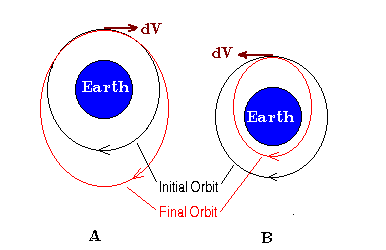The left side of the figure (A) shows the orbital change
that results from a velocity increment component along the
direction of the satellite track. The originally circular
orbit becomes an ellipse with a perigee equal to the circular
radius, and a larger apogee. In (B) the velocity component
is in the direction opposite to the satellite motion. The
orbit becomes elliptical, with the apogee now equal to the
circular radius, and a smaller perigee.

The perigee (q) is the closest distance of the orbit to the Earth and the apogee (p) is the furthest distance of the orbit from the Earth. The sum of the two is twice the semi-major axis (a) of the ellipse. The perigee and apogee are also related to the semi-major axis by the eccentricity (e) of the ellipse:

p = a ( 1 + e )
q = a ( 1 - e)
p + q = 2 a
Given the velocity (vc) and radius (rc) of an initial assumed circular orbit, as well as a tangential velocity component δv, we can compute the size parameters and the period of the new orbit. We need to consider the positive (along track) and negative (opposing track) velocity increments separately.
POSITIVE VELOCITY INCREMENT

a = GM / ( vc2 - 2 vc δv )
q = rc
e = 1 - rc / a
p = a ( 1 + e )
T = 2 π √( a3 / GM )

NEGATIVE VELOCITY INCREMENT

a = GM / ( vc2 + 2 vc δv )
(Note that δv is the magnitude of the velocity increment)
p = rc
e = ( rc / a ) -1
q = a ( 1 - e )
T = 2 π √( a3 / GM )

The period of the original circular orbit is:
T = 2 π √( rc3 / GM )

The following tables give an idea of the magnitude of orbital changes that may occur as a function of increments velocity.

```ORBIT CHANGE - delta V along track positive

Initial      V=50m/s    V=100m/s    V=150m/s    V=200m/s    V=250m/s
Ht   Per    Ht   Per    Ht   Per    Ht   Per    Ht   Per    Ht   Per
200  88.4   371  90.1   546  91.9   726  93.7   911  95.6  1102  97.6
300  90.4   475  92.2   654  94.0   839  95.9  1028  97.9  1223  99.9
400  92.4   579  94.3   762  96.1   951  98.1  1145 100.1  1344 102.2
500  94.5   683  96.4   871  98.3  1063 100.3  1262 102.4  1465 104.6
600  96.5   787  98.5   979 100.5  1176 102.6  1379 104.7  1587 107.0
700  98.6   891 100.6  1087 102.7  1289 104.8  1496 107.1  1709 109.4
800 100.7   995 102.8  1195 104.9  1401 107.1  1613 109.4  1831 111.8
900 102.8  1099 105.0  1304 107.1  1514 109.4  1730 111.8  1953 114.2
1000 105.0  1203 107.1  1412 109.4  1627 111.7  1848 114.2  2075 116.7
1100 107.1  1307 109.3  1521 111.7  1740 114.1  1966 116.6  2198 119.1
1200 109.3  1412 111.6  1629 113.9  1853 116.4  2083 119.0  2321 121.6

ORBIT CHANGE - delta V along track negative

Initial      V=50m/s    V=100m/s    V=150m/s    V=200m/s    V=250m/s
Ht   Per    Ht   Per    Ht   Per    Ht   Per    Ht   Per    Ht   Per
200  88.4    33  86.7  -129  85.1  -287  83.5  -442  82.0  -593  80.5
300  90.4   130  88.6   -36  87.0  -198  85.4  -356  83.8  -511  82.3
400  92.4   226  90.6    56  88.9  -110  87.2  -271  85.6  -429  84.1
500  94.5   322  92.6   148  90.9   -21  89.2  -186  87.5  -347  85.9
600  96.5   418  94.7   241  92.8    68  91.1  -100  89.4  -265  87.7
700  98.6   514  96.7   333  94.8   157  93.0   -15  91.2  -183  89.5
800 100.7   610  98.7   425  96.8   245  94.9    70  93.1  -101  91.4
900 102.8   706 100.8   518  98.8   334  96.9   155  95.0   -20  93.2
1000 105.0   802 102.9   610 100.8   422  98.9   239  96.9    61  95.1
1100 107.1   898 104.9   702 102.9   511 100.8   324  98.9   143  97.0
1200 109.3   994 107.0   794 104.9   599 102.8   409 100.8   224  98.9

```
The first table is for positive velocity increments, and the second is for negative velocity increments, both along the original orbital path. The first two columns give the height(km) and period(minutes) of an initial circular orbit. The next five sets of two columns give the same quantities (height and period) for different incremental velocity changes. In the positive increment case, the height is the apogee of the ellipse, the perigee being equal to the circular height. In the the negative case, the height is the perigee of the ellipse, the apogee being equal to the circular height.

A negative value for the perigee means that it is below the surface of the Earth. A fragment traversing such an ellipse will obviously not complete one orbit, but will either burn up on re-entry or hit the Earth within less than one-half of the orbital period. (Even were the fragment to hit the Earth, only a fraction of its initial mass would survive ablation travelling through the atmosphere).

Velocity increments out of the original orbital plane tend not to change the shape of the orbit significantly but do change its orientation in space. In particular, the inclination of the orbit may be changed. The change depends on where the velocity increment occurs. The greatest change to inclination occurs when the velocity increment occurs around the equator, and is least when the sub-latitude of the target is near its inclination value (positive or negative).

Gabbard Diagrams

John Gabbard developed a diagram, named after him, that is very useful for illustrating the orbital changes we have just been discussing. A Gabbard diagram is a scatter plot of height versus period. The apogee and perigee of each ejected fragment is shown as a point on the diagram. The resultant plot looks like two asymmetrical boomerangs joined at their apices. An example is shown below: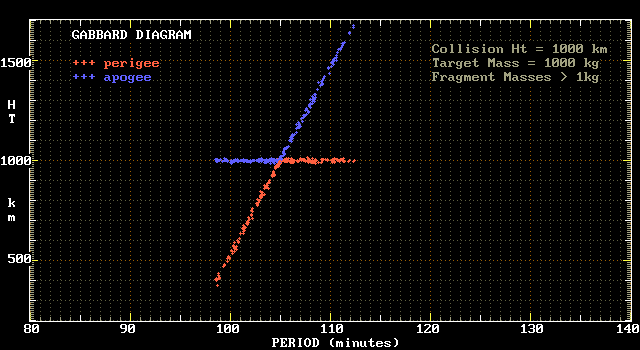The data used to make this plot is the data in the aforementioned example of a 1000 kg target. Only mass fragments 1 kilogram and larger have been used to compile the diagram. The catastrophic collision is assumed to have taken place at an altitude of 1000 km. The point where the two lines intersect is the original circular orbit at an altitude of 1000 km and with a period of 105 minutes. Points to the right of this location represent fragments that have been scattered in the forward direction of motion, while points to the left represent fragments scattered opposite the initial target motion. Note how the perigee heights (red points) in the first case become the apogee heights (blue points) in the second.

The next diagram illustrates how the smaller fragments are scattered into larger and smaller orbits than are their more massive counterparts.In this case apogee (+) and perigee (-) points are distinguished by different symbols, and the different colours are used to indicate different masses. It can be seen that the largest masses (≥100 kg) are relatively close to the original orbit, whereas the smallest masses (≥1kg) are the most dispersed.

Debris Orbital Decay

Gabbard diagrams are also useful for indicating the orbital decay of debris fragments. We have already seen in one of the tables above that when a collision occurs at a sufficiently low altitude some of the fragments that are ejected in the opposite direction to the original motion will be forced into orbits with a perigee below the Earth's surface. These will undergo atmospheric entry in less than half the period of their orbit. Because most of these particles will be those of smaller mass, they will in general burn up during reentry. Heavier fragments that may have a chance of depositing at least some of their mass on the Earth's surface will generally be the last fragments to decay due to atmospheric drag.

It is not necessary for a fragment to have a negative perigee to survive less than one orbit. Any fragment with a perigee of less than about 100 km will encounter so much air resistance (drag) that it will never make it back to apogee, and will deorbit in less than one period.

Fragments above this height will lose energy, mostly at perigee, and this will reduce their apogee height. Interestingly enough, their perigee height changes a lot less, until the apogee is brought down close to the perigee. The lower the perigee height of a fragment the larger the reduction in apogee height. This is seen on a Gabbard diagram as a drooping of the apogee plot at the left of the diagram.

The following two diagrams illustrate this decay. The first diagram is a plot within one orbit of the collision, which this time has taken place at a height of 300 km.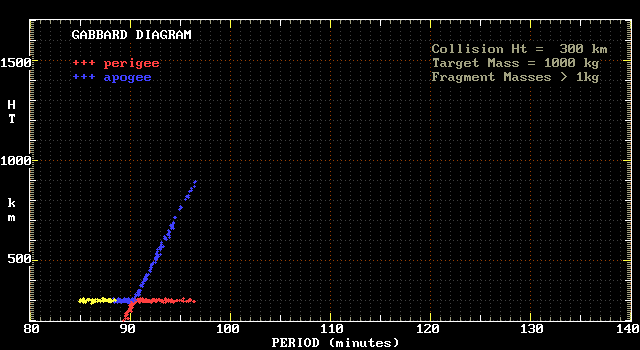The yellow points represent those fragments with perigees below 100 km, and which are thus not present after one orbit.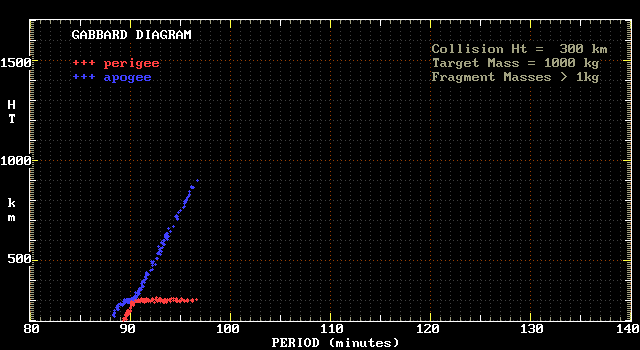Note the progressive droop in apogee as the period gets smaller. This plot simulates the fragment population some days after the collision, in conditions of sunspot minimum (ie minimal solar X-ray and shortwave UV output, which implies low atmospheric densities in the upper atmosphere).